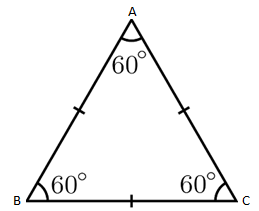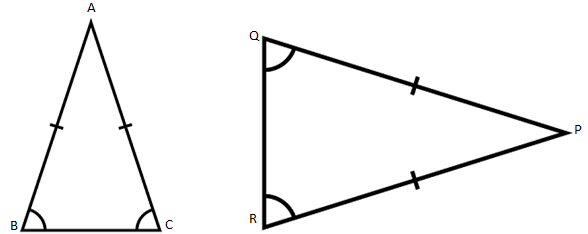# Isosceles Triangle, Equilateral Triangle, Scalene Triangle

A triangle is a three-sided polygon, which has 3 vertices and 3 sides enclosing 3 angles. The sum of interior angles in a triangle is 180 degrees. Here we will be introduced with the types of triangle on the basis of the length of their sides.

There are three type of triangles based on the sides.

(i) Equilateral Triangle

(ii) Isosceles triangle

(iii) Scalene Triangle

## What is Equilateral Triangle?An equilateral triangle is the one in which all the three sides are equal. It is a special case of the isosceles triangle where the third side is also equal. In the triangle ABC, AB = BC = CA. Properties of an equilateral triangle are:

• It has 3 equal sides.
• It has 3 equal angles.
• Since the sum of the interior angles is 180 degrees, every angle of an equilateral triangle is 60 degrees.
• It is a regular polygon with 3 sides.
• The altitude and median from a vertex is the same single line.
• The ortho-centre and centroid of the triangle is the same point.

## What is Isosceles Triangle?An isosceles triangle is a triangle which comprises 2 equal sides, regardless of the direction of the apex of the triangle points. Isosceles triangles have their application in determining the angles. In the isosceles triangle ABC, AB = AC. Similarly, in triangle PQR, PQ = PR. Vertices A and P are known as peaks and BC and QR (unequal sides) are known as the base of the isosceles triangle. Some of its properties are:

• It has two equal sides.
• It has two equal angles, that is, the base angles.
• When the third angle is 90 degrees, it is called a right isosceles triangle.
• The altitude from the apex to the base bisects the angle at the apex.

## What is Scalene Triangle?A Scalene Triangle is a one in which all the three sides of a triangle are of different length (unequal length). Triangle ABC (shown above) is a scalene triangle.

Some of the properties of Scalene triangle is as follows-

• It has no equal sides, i.e. all the sides are unequal
• All the three angle are unequal, but the sum of all the angle is 180∘

### Video Lesson on Types of Triangles